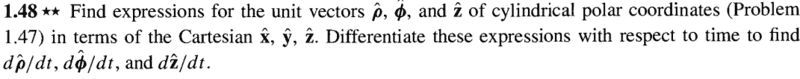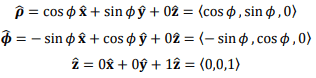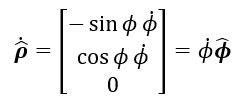# Cylindrical coordinates: unit vectors and time derivatives

## Homework Statement## The Attempt at a Solution

I have found expressions for the unit vectors for cylindrical coordinates in terms of unit vectors in rectangular coordinates.I have also found the time derivatives of the unit vectors in cylindrical coordinates. However, I am having trouble seeing how it simplifies. For instance, I do not understand how to arrive at the following for the rho hat unit vector.Can someone enlighten me, please?

## Answers and Replies

PeroK
Science Advisor
Homework Helper
Gold Member
2020 Award
You say you have the time derivatives of the unit vectors. But, if the derivative of ##\hat{\rho}## is not that given, then you must have made a mistake.

Chestermiller
Mentor

## Homework Statement

View attachment 237940

## The Attempt at a Solution

I have found expressions for the unit vectors for cylindrical coordinates in terms of unit vectors in rectangular coordinates.
View attachment 237941
I have also found the time derivatives of the unit vectors in cylindrical coordinates. However, I am having trouble seeing how it simplifies. For instance, I do not understand how to arrive at the following for the rho hat unit vector.
View attachment 237943
Can someone enlighten me, please?
You have $$\dot{\hat{\rho}}=(-\sin{\phi}\hat{x}+\cos{\phi}\hat{y})\dot{\phi}$$But you already showed that $$\hat{\phi}=(-\sin{\phi}\hat{x}+\cos{\phi}\hat{y})$$
Do you see how it works out now?

•Mason Smith
You have $$\dot{\hat{\rho}}=(-\sin{\phi}\hat{x}+\cos{\phi}\hat{y})\dot{\phi}$$But you already showed that $$\hat{\phi}=(-\sin{\phi}\hat{x}+\cos{\phi}\hat{y})$$
Do you see how it works out now?
That makes perfect sense. Thank you so much for the insight, Chestermiller!